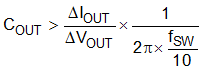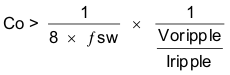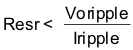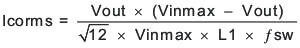SLVSDV8 July   2017

PRODUCTION DATA.

1. Features
2. Applications
3. Description
4. Revision History
5. Pin Configuration and Functions
6. Specifications
7. Detailed Description
1. 7.1 Overview
2. 7.2 Functional Block Diagram
3. 7.3 Feature Description
4. 7.4 Device Functional Modes
8. Application and Implementation
1. 8.1 Application Information
2. 8.2 Typical Application
1. 8.2.1 Design Requirements
2. 8.2.2 Detailed Design Procedure
3. 8.2.3 Application Curves
9. Power Supply Recommendations
10. 10Layout
11. 11Device and Documentation Support
12. 12Mechanical, Packaging, and Orderable Information

#### Package Options

Refer to the PDF data sheet for device specific package drawings

• RNV|18
• RNV|18

#### 8.2.2.4 Output Capacitor

There are two primary considerations for selecting the value of the output capacitor. The output voltage ripple and how the regulator responds to a large change in load current. The output capacitance needs to be selected based on the more stringent of these two criteria.

The desired response to a large change in the load current is the first criteria and is typically the most stringent. A regulator does not respond immediately to a large, fast increase or decrease in load current. The output capacitor supplies or absorbs charge until the regulator responds to the load step. The control loop needs to sense the change in the output voltage then adjust the peak switch current in response to the change in load. The minimum output capacitance is selected based on an estimate of the loop bandwidth. Typically the loop bandwidth is fSW/10. Equation 18 estimates the minimum output capacitance necessary, where ΔIOUT is the change in output current and ΔVOUT is the allowable change in the output voltage.

For this example, the transient load response is specified as a 4% change in VOUT for a load step of 2 A. Therefore, ΔIOUT is 2 A and ΔVOUT is 72 mV. Using these numbers gives a minimum capacitance of 63 μF. This value does not take the ESR of the output capacitor into account in the output voltage change. For ceramic capacitors, the effect of the ESR can be small enough to be ignored. Aluminum electrolytic and tantalum capacitors have higher ESR that must be considered for load step response.

Equation 19 calculates the minimum output capacitance needed to meet the output voltage ripple specification. Where fsw is the switching frequency, Vripple is the maximum allowable output voltage ripple, and Iripple is the inductor ripple current. In this case, the target maximum output voltage ripple is 9 mV. Under this requirement, Equation 19 yields 25 μF.

vertical spacer

Equation 18.vertical spacer

Equation 19.Where:

• ΔIOUT is the change in output current
• ΔVOUT is the allowable change in the output voltage
• fsw is the regulators switching frequency

vertical spacer

Equation 20 calculates the maximum combined ESR the output capacitors can have to meet the output voltage ripple specification and this shows the ESR should be less than 7 mΩ. In this case ceramic capacitors will be used and the ESR of ceramic capacitors is typically much less than 7 mΩ. Capacitors also have limits to the amount of ripple current they can handle without producing excess heat and failing. An output capacitor that can support the inductor ripple current must be specified. Capacitor datasheets specify the RMS (Root Mean Square) value of the maximum ripple current. Equation 21 can be used to calculate the RMS ripple current the output capacitor needs to support. For this application, Equation 21 yields 370 mA and the ceramic capacitors used in this design will have a ripple current rating much higher than this.

Equation 20.vertical spacer

Equation 21.X5R and X7R ceramic dielectrics or similar should be selected for power regulator capacitors because they have a high capacitance to volume ratio and are fairly stable over temperature. The output capacitor must also be selected with the DC bias and AC voltage derating taken into account. The derated capacitance value of a ceramic capacitor due to DC voltage bias and AC RMS voltage is usually found on the manufacturer's website. For this application example, one 100 μF 6.3 V 1210 X5R ceramic capacitor with 2 mΩ of ESR is used. The estimated capacitance after derating using the capacitor manufacturer's website is 80 µF.